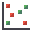The Rocscience International Conference 2021 Proceedings are now available. Read Now

# Scatter Plot

A Scatter Plot in RocSupport allows you to plot any two random variables from a Probabilistic Analysis, against each other on the same plot. Typically, the Scatter Plot is used to plot Factor of Safety versus an input data Random Variable (e.g. In-Situ Stress), to observe the correlation between the two.

To create a Scatter Plot:

1. Select Scatter Plotfrom the toolbar or the Statistics menu.
2. In the Scatter Plot dialog, select the data you would like to plot, on the Horizontal Axis and the Vertical Axis.
3. NOTE: in the list of variables to plot, the analysis output variables are listed first (e.g. Factor of Safety, Tunnel Convergence), followed by the input random variables which you have defined (e.g. In-Situ Stress, Young's Modulus).

4. You may also choose the following plotting options, using the check boxes provided. See the Plotting Options topic for details.
• Plot Regression Line
• Show Failed
5. Select OK to generate the Scatter Plot.
6. Once a plot is generated, there are various options available if you right-click the mouse on the plot. See below for details.

## Correlation Coefficient

The Correlation Coefficient indicates the degree of correlation between the two variables plotted. The Correlation Coefficient can vary between -1 and 1 where numbers close to zero indicate a poor correlation, and numbers close to 1 or -1 indicate a good correlation. Note that a negative correlation coefficient simply means that the slope of the best fit linear regression line is negative.

## Alpha and Beta

Alpha and beta represent the y-intercept and slope, respectively, of the best fit linear regression line to the scatter plot data. The display of the linear regression line can be toggled on or off, by selecting the Show Regression Line option in the right click menu, on a Scatter Plot.